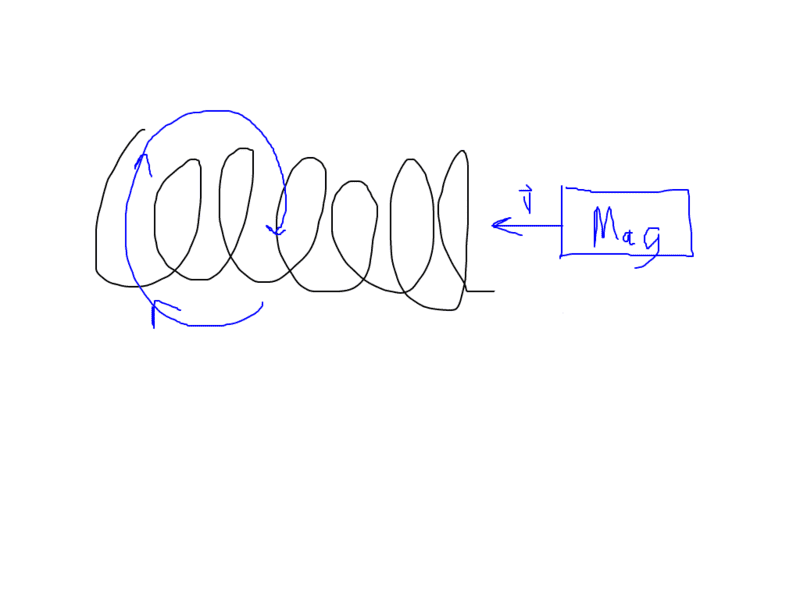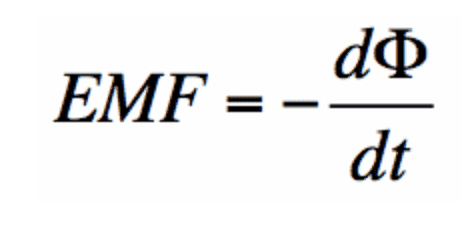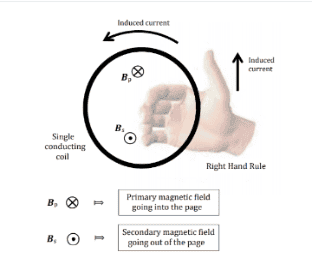# Lenz's Law and Faraday's Law clarification

• Marcin H

## Homework Statement

This isn't a problem, but I wanted to get some clarification of Lenz's law and Faradays law. So if you have a solenoid of wiresand you take a magnet and move it through the solenoid with a velocity v that magnet will induce a current into the wire according to the right hand rule. Thumb points in the direction of v and we curl our fingers around the solenoid. here is a super crappy picture:But according to faraday and current in a wire will produce a magnetic field that opposes the magnet and pushes it the other way. I understand that, but looking at the equation:I don't really understand how this tells us that the magnet will experience a force in the opposite direction. I don't fully understand what emf means besides that we can think about it as voltage and why it is equal to the negative of the time rate change of the flux. This is equation is saying that a changing magnetic field will induce an emf in our wire, but what is that emf? Does this mean we have an opposing current or something? Is emf the force that is pushing the magnet is the opposite direction that it is moving? if emf is induced in the wire, what does that mean exactly? voltage across a wire? Change in potential across a wire=0?? I feel like I don't have an intuitive sense of all of this...

## The Attempt at a Solution

check above

The EMF in the wire generates a current, and it can be quite large in the case of a short circuited solenoid, (i.e. with no series resistance in the loop). (The copper wire has a very low resistance). This current generates a magnetic field opposite the direction of the magnetic field of the magnet. Note: If the solenoid is open-circuited, any currents inside of it will be minimal. One additional note is the magnetic field from the currents in the wire reduce the change in the magnetic field from that of the magnet alone, so the EMF is not as strong, as it would be for an open-circuited solenoid.

•hutchphd
The EMF in the wire generates a current, and it can be quite large in the case of a short circuited solenoid, (i.e. with no series resistance in the loop). This current generates a magnetic field opposite the direction of the magnetic field of the magnet. Note: If the solenoid is open-circuited, any currents inside of it will be minimal.
So do you have 2 currents in the wire at the same time or something? One from the moving magnet and the emf?

As I recall, it is not Faraday's Law that predicts the opposing magnetic field but Ampere's Law. Faraday's Law tells us that a changing magnetic field creates an electric field, which gives an EMF. Ampere's Law tells us that a current or a changing electric field generates a magnetic field.

So we use Faraday's Law to predict that moving the magnet through the coil will generate a current in the coil. Then we use Ampere's Law to predict that that current will generate a magnetic field that pushes back against the magnet.

Lenz's Law is what we get when we put the two together - moving the magnet through the coil will induce a current that creates a magnetic field that pushes back.

With a moving magnet, the result is a change in the magnetic field. This causes the EMF by Faraday's law. Magnetic attraction of the electrons is not responsible for any currents of any significance. The EMF is what generates the currents, but the solenoid needs to be in a closed circuit, even short-circuited, for maximum current generation. ## \\ ## The magnetic field that gets generated is in the opposite direction. I believe the EMF that gets generated is maximized just before the magnet goes inside the solenoid. This is when the non-uniform magnetic field from the magnet is causing the greatest rate of change in the solenoid as a function of time. By Lenz's law, the currents generate a magnetic field that are opposite the magnetic field of the magnet, and the problem is analogous to two magnets with like poles facing each other=they repel each other.

Last edited:
moving the magnet through the coil will induce a current that creates a magnetic field that pushes back.

So is the picture i drew (very poorly) up there inccorect? If we move the magnet to the left through the coil, which direction will the current be? I thought that we take our right hand and point our thumb in the direction of the velocity and our fingers curl around the wire giving us the magnetic field. But that current does not produce a field that opposes the magnet right? In my drawing the current would have to flow the opposite way (blue line) to produce a magnetic field to oppose the magnet no?

With a moving magnet, the result is a change in the magnetic field. This causes the EMF by Faraday's law. Magnetic attraction of the electrons is not responsible for any currents of any significance. The EMF is what generates the currents, but the solenoid needs to be in a closed circuit, even short-circuited, for maximum current generation.
I'm still not following. so if a changing magnetic field induces and emf then that is the only current in the wire? and that current will flow in a direction such that that current will produce a magnetic field that will oppose the magnet. So the current I drew in my picture is incorrect? how does the right hand rule work for that. if we have a current and want to find the direction of the magnetic field. Thumb in direction of current and the curl is the magnetic field that is produced right? So the picture i drew is incorrect?

I'm still not following. so if a changing magnetic field induces and emf then that is the only current in the wire? and that current will flow in a direction such that that current will produce a magnetic field that will oppose the magnet. So the current I drew in my picture is incorrect? how does the right hand rule work for that. if we have a current and want to find the direction of the magnetic field. Thumb in direction of current and the curl is the magnetic field that is produced right? So the picture i drew is incorrect?
Yes, the currents need to circulate the other way in the solenoid. Also, see my edited post 5 above. The way I like to remember the direction of current is that for current into the paper, the magnetic field on the right side points downward. (I do not use a right hand rule for this.) ## \\ ## Note: The magnetic field from the magnet is assumed to have its + pole in front=i.e. in the direction of motion. The magnetic field from the magnet points nearly straight ahead and is stronger as you get closer to the endface of the magnet. ## \\ ## Note: The magnet is not a moving charge. It appears you might have tried to model the magnetic field from it by assuming some kind of right hand rule for it. (If this part is not the case, simply ignore). Anyway, this does not apply here. ## \\ ## What you need to look at is what is ## d \Phi/dt ## as the magnet approaches the solenoid. ## B ## is getting stronger, (more positive), assuming the + pole of the moving magnet is in front.

Last edited:
So is the picture i drew (very poorly) up there inccorect? If we move the magnet to the left through the coil, which direction will the current be? I thought that we take our right hand and point our thumb in the direction of the velocity and our fingers curl around the wire giving us the magnetic field. But that current does not produce a field that opposes the magnet right? In my drawing the current would have to flow the opposite way (blue line) to produce a magnetic field to oppose the magnet no?
A practical way to get the current direction is by Fleming's Right Hand Rule for generators. Focus on a point at the top of the coil above the North pole of the magnet. It is moving right relative to the magnetic field of the magnet. The magnetic field lines there are pointing partly upwards - they come out of the left (North) end of the magnet and curve upwards before looping back around to come back in the right (South) end. Using Fleming's rule, we see that, in the coils above the North end, this induces a current towards the viewer, which is the opposite direction from what is shown.

We then use Fleming's left-hand rule for motors to see that, with the current and mag field direction as above, a force pushes the coil to the left. That force comes from the North end of the magnet so, by Newton's law, it pushes back against the North end of the magnet in the rightwards direction.

The same thing happens at the South pole of the magnet. Above that a current is induced in the opposite direction, which is the direction shown in your diagram, in the coil near that pole. Applying the left-hand rule we then see that the part of the coil above the South pole pushes the South pole to the right.

•In "the thumb pointing in the direction of v" of the right hand rule of Ampere's Law, "v" refers to the direction of the moving electrons in the conductor where the induced current is produced. The "v" does not refer to the velocity of the magnet approaching the conductor. If the magnet remains stationary (the "v" of the magnet is zero), the magnetic flux it produces in the conductor does not change so no induced current is produced. But if the magnet moves toward the conductor, the magnetic field experienced by the conductor becomes stronger and that is what changes the magnetic flux through the conductor that induces an electric current. Since an electric current flows in the direction of decreasing electric potential V, that decrease ΔV in the electric potential of the previously equipotential surface of the conductor due to the change in the magnetic flux is what is called an induced EMF. An EMF is actually not a force (vector) but a change ΔV in electric potential (scalar). I think many people would agree that EMF is a misnomer for ΔV.
Also, to understand Faraday's law properly, you have to realize that the law actually refers to two magnetic fields; a primary magnetic field carried by a magnet moving relative to the conductor and a secondary magnetic field produced in the conductor by the induced current.
One other thing. Since a solenoid consists of a coil with many turns, it would simplify your understanding if you think first of just one coil only. Suppose a circular coil is on the plane of this page. If a bar magnet coming above the page approaches the coil on the page, the downward motion of the magnet causes the conducting coil to experience an increasing primary magnetic field. This increases the flux Φ of the primary field through the conductor. Since the magnet is coming from above, the increase in Φ through the coil is due to longer primary magnetic field vectors coming from the top and passing downward through the coil. According to Lenz's law, the induced current appears in such a way as to oppose the change in Φ. And as Ampere's law says, this induced current must be also due to a magnetic field. Since a stationary magnet with its own constant primary field does not produce an induced current in the conductor while that same magnet with constant magnetic field moving relative to the conductor now produces an induced current, Ampere's law says that a second magnetic field, which could be called a secondary magnetic field to distinguish it from that carried by the magnet, must now be present also (see attached file).
Adding all the secondary magnetlc fields produced in each coil, we get the total magnetic field produced by the solenoid.

#### Attachments

• Lenz1.pdf
113.1 KB · Views: 51
Your picture is incomplete because it does not show the direction of the magnetic field lines generated by the bar magnet. Say the North pole of the magnet is facing the solenoid. The quick way to see what's going on is through Lenz's law and by viewing the solenoid as equivalent to a bar magnet with a North and South pole.
1. The magnetic field lines from the bar magnet point to the left.
2. Moving the bar magnet to the left would tend to increase the flux through the solenoid.
3. The induced current in the solenoid would be in a direction that will oppose the proposed increase of flux lines to the left, i.e. the induced magnetic field lines would point to the right.
4. This means that the North pole of the solenoid is facing the North pole of the bar magnet.
5. Magnets with North poles facing each other repel.

I admit that it was a negligemce on my part that I did not specifically indicate the north and south poles of the bar magnet and the directions of its magnetic field but they should be self explanatory in the attachment which I included in my reply that I posted in #10. Here it is again with some little modifications.I considered only one coil of the solenoid and not all the coils taken together at one time. I think that would greatly simplify the understanding. I also looked at the coil from the top directly above the plane containing it. With the bar magnet moving towards the coil and coming directly from above, the cross inside a circle ⊗ representing the the primary magnetic field ##B_{\rm{p}} produced by the bar magnet should be understood as a view of the tail of the primary field vector. That could only mean that the north pole of the bar magnet were moving towards the coil because by convention, magnetic field lines originate from the north pole and go back to the south pole. If it were the south pole moving towards the coil, then one would see the head of a vector instead, which is frequently represented by a dot inside a circle, something like this symbol Θ.
Then, to oppose the increase in flux due to ⊗ moving downward, magnetic field vectors Θ moving upward must appear, and they could come only from an induced current moving counter clockwise in the conducting coil, according to Ampere's law. So piling up a number of such identical coils one directly on top of another, all having the same magnetic field Θ pointing upward, then we get a solenoid. With magnetc field lines always originating from the noth pole of magnets, the Θ and ⊗ facing each other means that we have the north poles of two magnets also facing one another mutually exerting repulsive force, exactly like what you said earlier.